• 2021-11-15 19:04:14

分类是一种重要的数据分析形式，它提取刻画重要数据类的模型。数据分类是一个两阶段过程，包括学习阶段（构建分类模型）和分类阶段（使用模型预测给定数据的类标号）。
线性分类： 指存在一个线性方程可以把待分类数据分开，或者说用一个超平面能将正负样本区分开，表达式为 y = w ⋅ x y=\pmb{w}\cdot\pmb{x} ，这里先说一下超平面，对于二维的情况，可以理解为一条直线，如一次函数。它的分类算法是基于一个线性的预测函数，决策的边界是平的，比如直线和平面。一般的方法有感知器，最小二乘法。
非线性分类： 指不存在一个线性分类方程把数据分开，它的分类界面没有限制，可以是一个曲面，或者是多个超平面的组合。

对于分类任务，线性回归模型就无能为力了，但是我们可以在线性模型的函数进行后再加入一层激活函数，这个函数是非线性的，激活函数的反函数叫做链接函数。我们有两种线性分类的方式：

1. 硬分类，我们直接需要输出观测对应的分类。这类模型的代表为：
• 线性判别分析（Fisher 判别）
• 感知机（Perceptron）
2. 软分类，产生不同类别的概率，这类算法根据概率方法的不同分为两种
• 生成式（根据贝叶斯定理先计算参数后验，再进行推断）：高斯判别分析（GDA）和朴素贝叶斯等为代表
1. Gaussian Discriminate Analysis
2. Naive Bayes
• 判别式（直接对条件概率进行建模）：Logistic Regression

## 1 介绍

感知机（Perceptron）是二分类的线性分类模型，其输入为实例的特征向量，输出为实例的类别，取+1和-1。感知机对应于输入空间(特征空间)中将实例划为正负两类的分离超平面，属于判别模型。感知机预测是用学习得到的感知机模型对新的输入实例进行分类，是神经网络与支持向量机的基础。## 2 感知机模型

### 2.1 函数模型

假设输入空间（特征空间）是 X ⊆ R p \mathcal{X} \subseteq \mathbb{R}^{p} ，，输出空间是 Y = { + 1 , − 1 } \mathcal{Y}=\{+1, -1\} ，有一可以被线性分类的样本集 T = { ( x i , y i ) } i = 1 N T = \{(\pmb{x}_{i},y_{i})\}_{i=1}^{N} ，其中 x i ∈ X \pmb{x}_{i}\in \mathcal{X} ，表示实例的特征向量，对应于输入空间（特征空间）的点；输出 y i ∈ Y \pmb{y}_{i}\in \mathcal{Y} 表示实例的类别。由输入空间到输出空间的如下函数：
f ( x ) = s i g n ( w 1 x i 1 + w 2 x i 2 + ⋯ + w p x i p + b ) = s i g n ( w T x + b ) (2-1) \begin{aligned} f(\pmb{x}) &= sign(w_1x_{i1}+w_{2}x_{i2}+\cdots+w_px_{ip}+b) \\&= sign(\pmb{w}^{T}\pmb{x}+b) \end{aligned} \tag{2-1}
称为感知机。其中 w \pmb{w} b b 为感知机模型参数， w ∈ R p \pmb{w} \in \mathbb{R}^{p} 叫做权值(weight)或权值向量(weight vector)， b b 叫作偏置(bias)， w ⋅ x \pmb{w} \cdot \pmb{x} 表示 w \pmb{w} x \pmb{x} 的内积，由内积的运算可知 w ⋅ x = w T x \pmb{w} \cdot \pmb{x}=\pmb{w}^{T}\pmb{x} sign是符号函数，即：
s i g n ( a ) = { + 1 a ≥ 0 − 1 a < 0 (2-2) sign(a)=\left\{\begin{matrix}+1\quad a\ge0\\-1 \quad a\lt0\end{matrix}\right. \tag{2-2}

感知机模型的假设函数为 f ( x ) f(\pmb{x}) ，通过输入特征向量 x \pmb{x} 即可判断其所属类别；模型的假设空间是定义在特征空间中的线性分类模型（linear classification moedl）或线性分类器（linear classifier），即函数集合 { f ∣ f ( x ) = w T x + b } \left \{ f \mid f(\pmb{x})= \pmb{w}^T\pmb{x}+b \right \}

### 2.2 几何解释

线性方程： w T x + b = 0 \pmb{w}^T\pmb{x} + b = 0 对应于特征空间 R p \mathbb{R}^{p} 中的一个超平面 S S ，其中 w \pmb{w} 是从超平面的法向量， b b 是超平面的截距。这个超平面将特征空间划分为两个部分。位于两部分的点（特征向量）分别被分为正、负两类。因此，超平面 S S 成为分离超平面（separating hyperplane），如图1.1所示。图1.1 感知机模型

在这里为什么 w \pmb{w} 是超平面法向量？
证明：超平面为 w T x + b = 0 \pmb{w}^T\pmb{x}+b=0 ，设超平面上两个点 x 1 ， x 2 \pmb{x}_1，\pmb{x}_2 ，则有
{ w T x 1 + b = 0 , ① w T x 2 + b = 0 , ② (2-3) \begin{cases} \pmb{w}^T\pmb{x}_1 + b =0, & \text{①} \\ \pmb{w}^T \pmb{x}_2 + b =0, & \text{②} \end{cases} \tag{2-3}
由 ① - ② 可得：
w T ( x 1 − x 2 ) = 0 (2-4) \pmb{w}^T(\pmb{x}_1 - \pmb{x}_2) =0 \tag{2-4}
由于 x 1 − x 2 \pmb{x}_1 - \pmb{x}_2 仍是平面上的点，而 对于任意 x 1 ， x 2 \pmb{x}_1，\pmb{x}_2 ，式子都成立，所以 w \pmb{w} 是超平面法向量。

x i ∈ R p , y i { − 1 , + 1 } (2-5) \pmb{x}_{i}\in \mathbb{R}^{p},y_{i}\left \{-1,+1\right \} \tag{2-5}

感知机算法使用随机梯度下降法（SGD）来在特征空间 R p \mathbb{R}^{p} 寻找一个超平面 w T x + b = 0 w^{T}x+b=0 来将数据划分为正、负两类，其中 w ∈ R p w\in \mathbb{R}^{p} ，是超平面的法向量。

超平面是纯粹的数学概念，不是物理概念，它是平面中的直线、空间中的平面的推广，只有当维度大于3，才称为“超”平面。通常，在1维空间里超平面为数轴上的一个点，在2维空间中的超平面是一条线，在3维空间中的超平面是一个平面。一个超平面可以将它所在的空间分为两半, 它的法向量指向的那一半对应的一面是它的正面, 另一面则是它的反面。

## 3 感知机学习策略

### 3.1 数据集的线性可分

给定数据集 T = { ( x 1 , y 1 ) , ( x 2 , y 2 ) , ⋯   , ( x N , y N ) } T=\left\{\left(\pmb{x}_{1}, y_{1}\right),\left(\pmb{x}_{2}, y_{2}\right), \cdots,\left(\pmb{x}_{N}, y_{N}\right)\right\} ，其中 x i ∈ X = R p x_{i} \in \mathcal{X}=\mathbb{R}^{p} y i ∈ Y = { + 1 , − 1 } ， i = 1 , 2 , ⋯   , N y_{i} \in \mathcal{Y}=\{+1,-1\}，i=1,2, \cdots, N ，如果存在某个超平面 S S 满足：
w T x + b = 0 \pmb{w}^T\pmb{x} + b =0

能将数据集的正实例点和负实例点完全正确地划分到超平面的两侧，即对所有 y i = + 1 y_i=+1 的实例 x i \pmb{x}_i ，有 w T x i + b > 0 \pmb{w}^T\pmb{x}_i + b > 0 ；对所有 y i = − 1 y_i=-1 的实例 x i \pmb{x}_i ，有 w T x i + b < 0 \pmb{w}^T\pmb{x}_i + b <0 ，则称数据集 T T 为线性可分数据集；否则，称数据集 T T 线性不可分。
在学习感知机模型中，需要确保数据集线性可分，才能够找到一个完全将正实例点和负实例点正确分开的分离超平面，即才能够确定感知机模型参数 w ， b \pmb{w}，b

### 3.2 损失函数

假设训练数据集是线性可分的，感知机学习的目的是求得一个能够将训练集整实例点和负实例点完全正确分开的分离超平面。为了找出这样的超平面，即确定感知机模型参数 w \pmb{w} b b , 需要确定一个学习策略，即定义（经验）损失函数并将损失函数极小化。

损失函数的一个自然选择是误分类点的个数，即 L ( w ) = ∑ i = 1 N I { − y i ( w T x i + b ) > 0 } L(\pmb{w})=\sum_{i=1}^{N}I\left \{-y_{i}(\pmb{w}^{T}\pmb{x}_{i}+b)>0\right \} ，但是这样的损失函数是不可导的，不易优化。因此采用另一种损失函数，即误分类点到超平面的总距离。

感知机的思想是错误驱动。对于误分类的数据 ( x i , y i ) (x_{i},y_{i}) 来说，因为当 w T x i + b > 0 \pmb{w}^T\pmb{x}_i+b > 0 时， y i = − 1 y_i=-1 ，而当 w T x i + b < 0 \pmb{w}^T\pmb{x}_i+b < 0 时， y i = 1 y_i=1 ，所以满足以下关系：
− y i ( w T x i + b ) > 0 (3-1) {-y_{i}(\pmb{w}^{T}\pmb{x}_{i}+b)}>0 \tag{3-1}

由点到线的距离公式可知，我们设直线 L L 方程为 A x + B y + C = 0 Ax+By+C=0 ，点 P P ( x 0 , y 0 ) (x_0,y_0) ，则点 P P 到直线 L L 的距离为：
d = ∣ A x 0 + B y 0 + C ∣ A 2 + B 2 (3-2) {\large d=\frac{|Ax_{0}+By_{0}+C|}{\sqrt{A^{2}+B^{2}}}} \tag{3-2}

设输入空间上任意一点 x \pmb{x} 到超平面 S S 的距离，也就是在超平面法向量 w \pmb{w} 上的投影长度，在超平面上取一点 x ′ x'
d = ∣ w T ( x − x ′ ) ∣ ∣ ∣ w ∣ ∣ = ∣ w T x + b ∣ ∣ ∣ w ∣ ∣ (3-3) d=\frac{|\pmb{w}^T(\pmb{x}-\pmb{x}')|}{||\pmb{w}||}=\frac{|\pmb{w}^T\pmb{x}+b|}{||\pmb{w}||} \tag{3-3}

误分类点 x i \pmb{x}_i 到超平面 S S 的距离是
− 1 ∣ ∣ w ∣ ∣ y i ( w T x i + b ) (3-4) -\frac{1}{||\pmb{w}||} y_i(\pmb{w}^T \pmb{x}_i + b) \tag{3-4}
假设超平面 S S 的误分类点集合为 M M ，那么所有误分类点到超平面 S S 的总距离为
− 1 ∣ ∣ w ∣ ∣ ∑ x i ∈ M y i ( w T x i + b ) (3-5) -\frac{1}{||\pmb{w}||} \sum_{\boldsymbol{x}_i \in M} y_i (\pmb{w}^T \pmb{x}_i + b) \tag{3-5}

不考虑 1 ∣ ∣ w ∣ ∣ \dfrac{1}{||\boldsymbol{w}||} ，就得到感知机学习的损失函数。

感知机 s i g n ( w T x + b ) sign(\pmb{w}^{T}\pmb{x}+b) 学习的损失函数定义为
L ( w , b ) = − ∑ x i ∈ M y i ( w T x i + b ) (3-6) L(\pmb{w}, b) = -\sum_{\boldsymbol{x}_i \in M} y_i (\pmb{w}^T \pmb{x}_i + b) \tag{3-6}

由上面的式子，我们可以看到，损失函数是非负的。如果没有误分类点，损失函数值是0；误分类点越少，误分类点距离超平面越近，损失函数值越小。一个特定的样本点的损失函数，在误分类时是参数 w , b \pmb{w}, b 的线性函数，在正确分类时是0。因此，给定训练数据集 T T ， 损失函数 L ( w , b ) L(\pmb{w}, b) w , b \pmb{w}, b 的连续可导函数。损失函数的优化目标，就是期望使误分类的所有样本，到超平面的距离之和最小。

感知机学习的策略是在假设空间中选取使损失函数式（3-6）最小的模型参数 w , b \pmb{w}, b ，即感知机模型。

在空间里，向量可以看做是一个点（以原点为起始点的向量），对于分离超平面方程里的向量 x \pmb{x} ，就可以看做由坐标原点到超平面任意“点”的向量。

## 4 感知机学习算法

### 4.1 原始形式

感知机学习算法是误分类驱动的，具体采用随机梯度下降法（ stochastic gradient descent）。首先，任意选取一个超平面 w 0 ， b 0 \pmb{w}_0，b_0 ，然后用梯度下降法不断地极小化目标函数（3-6）。极小化过程中不是一次使 M M 中所有误分类点的梯度下降，而是一次随机选取一个误分类点使其梯度下降。
假设误分类点集合 M M 是固定的，则损失函数 L ( w , b ) L(\pmb{w}, b) 的梯度为：
▽ w L ( w , b ) = ∂ L ( w , b ) ∂ w = − ∑ x i ∈ M y i x i ▽ b L ( w , b ) = ∂ L ( w , b ) ∂ b = − ∑ x i ∈ M y i (4-1) \bigtriangledown_\boldsymbol{w} L(\boldsymbol{w}, b) = \frac{\partial L(\boldsymbol{w},b)}{\partial \boldsymbol{w}}=-\sum _{\boldsymbol{x}_{i}\in M}y_{i}\boldsymbol{x}_{i} \\ \bigtriangledown_b L(\boldsymbol{w}, b) = \frac{\partial L(\boldsymbol{w},b)}{\partial b}=-\sum _{\boldsymbol{x}_{i}\in M}y_{i} \tag{4-1}

如果样本非常多的情况下，计算复杂度较高，但是，实际上我们并不需要绝对的损失函数下降的方向，我们只需要损失函数的期望值下降，但是计算期望需要知道真实的概率分布，我们实际只能根据训练数据抽样来估算这个概率分布（经验风险）：
E D [ E p ^ [ ∇ w L ( w ) ] ] = E D [ 1 N ∑ i = 1 N ∇ w L ( w ) ] \mathbb{E}_{\mathcal D}[\mathbb{E}_{\hat p}[\nabla_\boldsymbol{w}L(\boldsymbol{w})]]=\mathbb{E}_{\mathcal D}[\frac{1}{N}\sum\limits_{i=1}^N\nabla_\boldsymbol{w}L(\boldsymbol{w})]
我们知道， N N 越大，样本近似真实分布越准确，但是对于一个标准差为 σ \sigma 的数据，可以确定的标准差仅和 N \sqrt{N} 成反比，而计算速度却和 N N 成正比。因此可以每次使用较少样本，则在数学期望的意义上损失降低的同时，有可以提高计算速度，如果每次只使用一个错误样本，我们有下面的更新策略：
随机选取一个误分类点 ( x i , y i ) (\pmb{x}_i, y_i) ，对 w , b \pmb{w}, b 进行更新：
w ← w + η y i x i b ← w b + η y i (4-2) \boldsymbol{w}\leftarrow \boldsymbol{w}+\eta y_{i}\boldsymbol{x}_{i}\\ b\leftarrow\boldsymbol{w} b+\eta y_{i} \tag{4-2}

式中 η ( 0 < η ≤ 1 ) \eta (0 < \eta \leq 1) 是步长，在统计学习中又称为学习率（learning rate）。步长越长，下降越快，如果步长过长，会跨过极小点导致发散；如果步长过小，消耗时间会很长。通过迭代可以期待损失函数 L ( w , b ) L(\pmb{w}, b) 不断减小，直到为0。最终得到的超平面并不唯一，随着选取的初值不同或选取不同的误分类点，我们得到的超平面也不一定相同。

使用单个观测更新可以在一定程度上增加不确定度，从而减轻陷入局部最小的可能。

1. 感知机学习算法的原始形式

输入： T = { ( x 1 , y 1 ) , ( x 2 , y 2 ) , … , ( x N , y N ) } x i ∈ X = R p , y i ∈ Y = { − 1 , + 1 } , i = 1 , 2 , … , N ;    0 < η ≤ 1 T=\{(\pmb{x}_1,y_1),(\pmb{x}_2,y_2),\dots,(\pmb{x}_N,y_N)\}\quad \pmb{x}_i\in \mathcal X=\mathbb R^p , y_i\in \mathcal Y =\{-1,+1\}, i=1,2,\dots,N; \ \ 0<\eta\leq 1

输出： w , b ; f ( x ) = s i g n ( w T x + b ) \pmb{w},b;f(\pmb{x})=sign(\pmb{w}^T\pmb{x}+b)

1. 选取初值 w 0 , b 0 \pmb{w}_0,b_0

2. 训练集中选取数据 ( x i , y i ) (\pmb{x}_i,y_i)

3. 如果 y i ( w T x i + b ) ⩽ 0 y_i(\pmb{w}^T\pmb{x}_i+b)\leqslant 0
w ← w + η y i x i b ← b + η y i \pmb{w}\leftarrow \pmb{w}+\eta y_i\pmb{x}_i \\ b\leftarrow b+\eta y_i

4. 转至(2)，直至训练集中没有误分类点

这种学习算法直观上有如下解释： 当一个样本被误分类时，就调整 w \pmb{w} b b 的值，使超平面 S S 向误分类点的一侧移动，以减少该误分类点到超平面的距离，直至超平面越过该点使之被正确分类。
注意这个原始形式中的迭代公式，可以对 x i ​ \pmb{x}_i ​ 补1，将 w ​ \pmb{w}​ b ​ b​ 合并在一起，合在一起的这个叫做扩充权重向量，《统计学习方法》上有提到。

2. 算法的收敛性

为了便于叙述与推导，将偏置 b b 并入权重向量 w \pmb{w} ，记作 w ^ = ( w T , b ) T \hat{\pmb{w}}=(\pmb{w}^T, b)^T 同样也将输入向量加以扩充，加进常数 1，记作 x ^ = ( x T , 1 ) T \hat{\pmb{x}}=(\pmb{x}^T, 1)^T 这样， x ^ ∈ R p + 1 , w ^ ∈ R p + 1 \hat{x} \in \mathbb{R}^{p+1}, \hat{w} \in \mathbb{R}^{p+1} 。显然， w ^ ⋅ x ^ = w ⋅ x + b \hat{\pmb{w}} \cdot \hat{\pmb{x}}=\pmb{w} \cdot \pmb{x}+b

定理一 （Novikoff）：设训练数据集 T = { ( x 1 , y 1 ) , ( x 2 , y 2 ) , ⋯   , ( x N , y N ) } T=\left\{\left(\pmb{x}_{1}, y_{1}\right),\left(\pmb{x}_{2}, y_{2}\right), \cdots,\left(\pmb{x}_{N}, y_{N}\right)\right\} 是线性可分的，其中 x i ∈ X = R p x_{i} \in \mathcal{X}=\mathbb{R}^{p} y i ∈ Y = { + 1 , − 1 } ， i = 1 , 2 , ⋯   , N y_{i} \in \mathcal{Y}=\{+1,-1\}，i=1,2, \cdots, N ，则

（1）存在满足条件 ∣ w ^ o p t ∥ = 1 |\hat{\pmb{w}}_{\mathrm{opt}}\|=1 的超平面 w ^ o p t ⋅ x ^ = w o p t ⋅ x + b o p t = 0 \hat{\pmb{w}}_{\mathrm{opt}} \cdot \hat{\pmb{x}}=\pmb{w}_{\mathrm{opt}} \cdot \pmb{x}+b_{\mathrm{opt}}=0 将训练数据集完全正确分开；且存在 γ > 0 \gamma>0 ，对所有 i = 1 , 2 , ⋯   , N i=1,2, \cdots, N
y i ( w ^ o p t ⋅ x ^ i ) = y i ( w o p t ⋅ x i + b o p t ) ⩾ γ (4-3) y_{i}(\hat{\pmb{w}}_{\mathrm{opt}} \cdot \hat{\pmb{x}}_{i})=y_{i}(\pmb{w}_{\mathrm{opt}} \cdot \pmb{x}_{i}+b_{\mathrm{opt}}) \geqslant \gamma \tag{4-3}

（2）令 R = max ⁡ 1 ⩽ i ⩽ N ∥ x ^ i ∥ R=\max _{1 \leqslant i \leqslant N}\|\hat{\pmb{x}}_{i}\| ，则感知机算法在训练数据集上的误分类次数 k k 满足不等式
k ⩽ ( R γ ) 2 (4-4) k \leqslant\left(\frac{R}{\gamma}\right)^{2} \tag{4-4}

根据这两个定理可知，对于线性可分数据集，感知机学习算法原始形式收敛，即经过有限次迭代可以得到一个将训练数据集完全正确划分的分离超平面及感知机模型。Novikoff定理的证明过程可以参考：Convergence Proof for the Perceptron Algorithm

为什么采用随机梯度下降法而基于所有样本的梯度和均值的批量梯度下降法（BGD）是行不通的？主要在于我们的损失函数里面有限定，只有误分类的M集合里面的样本才能参与损失函数的优化。所以我们不能用最普通的批量梯度下降，只能采用随机梯度下降（SGD），但在较大规模的数据上，常用的是小批量随机梯度下降法。

3. 代码实现

import numpy as np
class Model:#定义感知机学习类
def __init__(self):
#构造时初始值化w = (0 ,0), b = 0
self.w = np.zeros(len(data) - 1,dtype=np.float32) #初始化参数 w , b,和学习率
self.b = 0
#学习率=1
self.l_rate = 100# self.data = data
def sign(self,x, w,b):
#定义感知机模型函数
y = np.dot(x,w) + b
return y
#随机梯度下降法
def fit(self, X_train, y_train):
is_wrong = False #判断是否有误分类点
while not is_wrong:
wrong_count = 0 #记录误分类点数目
for d in range( len( X_train) ):
x= X_train[d]
y = y_train[d]
#选取—个点进行判断
if y * self.sign(x, self.w,self.b)<=0: #判断是不是误分类点的标志
self.w = self.w + self.l_rate * np.dot(y,x)#更新参数
self.b = self.b + self.l_rate * y
wrong_count += 1 #误分类点数目加一
if wrong_count == 0:
is_wrong = True  #没有误分类点
return ' Perceptron Model! '


### 4.2 对偶形式

感知机算法对偶形式的目的是降低运算量，但是并不是在任何情况下都能降低运算量，而是在特征空间的维度很高时才能发挥作用。 每次感知机梯度下降算法的迭代都是选择一个误分类样本数据来更新 w 、 b \pmb{w}、b 参数。最终经过若干次的迭代后得到最终的结果。对于从来都没有误分类过的样本，它被选择参与 w 、 b \pmb{w}、b 迭代的次数是 0 0 ，假设样本点 ( x i , y i ) ∈ M (\pmb{x}_{i}, y_{i}) \in M 在迭代更新 w 、 b \pmb{w}、b 时被使用了 k i k_i 次，则 w 、 b \pmb{w}、b 关于 ( x i , y i ) ∈ M (\pmb{x}_{i}, y_{i}) \in M 的增量分别是 α i y i x i \alpha_i y_i\pmb{x}_i α i y i \alpha_i y_i α i = k i η ≥ 0 \alpha_i=k_i\eta \geq 0 ），因此在原始感知机算法中，算法最后收敛时的 w 、 b \pmb{w}、b 为：

w ← ∑ i = 1 N α i y i x i b ← ∑ i = 1 N α i y i (4-5) \begin{aligned} \pmb{w}& \leftarrow \sum\limits_{i=1}^N \alpha_iy_{i}\pmb{x}_{i}\\ b& \leftarrow \sum\limits_{i=1}^N \alpha_iy_{i} \end{aligned} \tag{4-5}

可以理解为从原始形式中的不断更新参数 w \pmb{w} b b 变成了学习某一点被误分类的次数 k i k_i （样本点 ( x i , y i ) ∈ M (\pmb{x}_{i}, y_{i}) \in M 由于误分类进行更新的次数）。
综上可以给出对偶形式下的参数迭代算法流程：

输入： T = { ( x 1 , y 1 ) , ( x 2 , y 2 ) , … , ( x N , y N ) } x i ∈ X = R p , y i ∈ Y = { − 1 , + 1 } , i = 1 , 2 , … , N ; 学 习 率 η （ 0 < η ≤ 1 ） T=\{(\pmb{x}_1,y_1),(\pmb{x}_2,y_2),\dots,(\pmb{x}_N,y_N)\}\quad \pmb{x}_i \in \mathcal{X}=\mathbb{R}^p , y_i \in \mathcal{Y} =\{-1,+1\}, i=1,2,\dots, N; 学习率 \eta（0< \eta \leq 1）

输出：
α , b ; f ( x ) = s i g n ( ∑ j = 1 N α j y j x j ⋅ x + b ) α = ( α 1 , α 2 , ⋯   , α N ) T \pmb{\alpha} ,b; f(\pmb{x})=sign\left(\sum_{j=1}^N\alpha_jy_j\pmb{x}_j\cdot \pmb{x}+b\right)\\ \pmb{\alpha}=(\alpha_1,\alpha_2,\cdots,\alpha_N)^T

1. α ← 0 , b ← 0 ​ \pmb{\alpha} \leftarrow 0,b\leftarrow 0​

2. 训练集中选取数据 ( x i , y i ) (\pmb{x}_i,y_i)

3. 如果 y i ( ∑ j = 1 N α j y j x j ⋅ x i + b ) ⩽ 0 ​ y_i\left(\sum_{j=1}^N\alpha_jy_j\pmb{x}_j\cdot \pmb{x}_i+b\right) \leqslant 0​
α i ← α i + η b ← b + η y i \alpha_i\leftarrow \alpha_i+\eta \\ b\leftarrow b+\eta y_i

4. 转至(2)，直至训练集中没有误分类点

其中 x i ⋅ x j \pmb{x}_{i}\cdot \pmb{x}_{j} 表示做内积运算，迭代更新至无误分类样本数据即可。在迭代更新时可以发现当某样本数据点在算法更新时使用次数越多，意味着它距离分离超平面越近，也就越难以正确分类，换言之，这类样本数据点对感知机算法学习的效果影响最大 。

1. Gram matrix
对偶形式中，训练实例仅以内积的形式出现。为了方便可预先将训练集中的实例间的内积计算出来并以矩阵的形式存储，这个矩阵就是所谓的Gram矩阵：
G = [ x i ⋅ x j ] N × N = [ x 1 ⋅ x 1 x 1 ⋅ x 2 … x 1 ⋅ x N x 2 ⋅ x 1 x 2 ⋅ x 2 … x 2 ⋅ x N … ⋯ … ⋯ x N ⋅ x 1 x N ⋅ x 1 … x N ⋅ x N ] (4-6) \pmb{G}=[\pmb{x}_i\cdot \pmb{x}_j]_{N\times N}=\left[\begin{array}{cccc} \pmb{x}_{1} \cdot \pmb{x}_{1} & \pmb{x}_{1} \cdot \pmb{x}_{2} & \ldots & \pmb{x}_{1} \cdot \pmb{x}_{N} \\ \pmb{x}_{2} \cdot \pmb{x}_{1} & \pmb{x}_{2} \cdot \pmb{x}_{2} & \ldots & \pmb{x}_{2} \cdot \pmb{x}_{N} \\ \ldots & \cdots & \ldots & \cdots \\ \pmb{x}_{N} \cdot \pmb{x}_{1} & \pmb{x}_{N} \cdot \pmb{x}_{1} & \ldots & \pmb{x}_{N} \cdot \pmb{x}_{N} \end{array}\right] \tag{4-6}

在对偶形式中引入了Gram矩阵来存储内积，可以提高运算速度， 而反观原始形式，每次参数改变，所有的矩阵计算全部需要计算，导致计算量比对偶形式要大很多， 这就是对偶形式的高效之处。

2. 代码实现

def fit( self,X,y):
#权重和偏置初始化
self.alpha = np.zeros ( X.shape)
self.b =0
train_complete_flag = False
Gram = np.dot(X, X.T) #存放样本两两内积的Gram矩阵（特点)
while not train_complete_flag:
error_count = 0
for i in range ( X.shape):
x_, y_ = X[i],y[i]
#有—个点当分类错误时,更新alpha_i和偏置
tmp_sum = np.dot(np.multiply(self.alpha,y),Gram[ :, i])
#进行误分类点判断
if y_* (tmp_sum+ self.b) <= 0:
self.alpha[i] += self.lr
self.b += self.lr * y_
error_count += 1
if not error_count:
train_complete_flag = True#训练完成后计算权重
self.w = np.dot(np.multiply (self.alpha,y),X)


## 5 训练过程

线性可分的训练过程：线性不可分的训练过程：## 6 总结

### 6.1 原始形式和对偶形式

1. 对比
假设特征空间是 R p \R^p ，样本数据为 N ​ N​ N ≪ p ​ N\ll p​ 。当考虑原始形式的感知机算法时，每轮迭代中至少要判断某个输入样本数据点是不是误分类点，既对于 ( x i , y i ) (\pmb{x}_{i},y_{i}) ，是否有   y i ( w T x i + b ) ⩽ 0   ​ \ y_{i}(\pmb{w}^T\pmb{x}_{i}+b)\leqslant 0\ ​ 。这里的运算量主要集中在求输入特征 x i \pmb{x}_{i} 和权值向量 w \pmb{w} 的内积上，时间复杂度为 O ( p ) ​ O(p)​ ，当特征空间维度非常高时，原始感知机算法效率很低；而在对偶形式的感知机算法中，对于输入数据点 ( x i , y i ) (\pmb{x}_{i},y_{i}) 是否为误分类点的条件转化为
y i ( ∑ j = 1 N α j y j x j ⋅ x i + b ) ⩽ 0 ​ y_i\left(\sum_{j=1}^N\alpha_jy_j\pmb{x}_j\cdot \pmb{x}_i+b\right) \leqslant 0​

注意到这里所有输入样本数据都仅仅以内积的形式出现，所以我们可以预先计算出输入样本数据两两之间的内积，得到所谓的 G r a m Gram 矩阵 G = [ ( x ( i ) , x ( j ) ) ] N × N ​ G=[(x^{(i)},x^{(j)})]_{N\times N}​ 。这样一来每次做误分类点检测时候只需要在 G r a m Gram 矩阵里查找就能获取内积 ( x i , x j ) (\pmb{x}_{i},\pmb{x}_{j}) ，所以这个误分类点检测的时间复杂度是 O ( 1 ) ​ O(1)​ 。也就是说，对偶形式的感知机算法，把每轮迭代的时间复杂度从特征空间维度 p ​ p​ 转移到了样本数据数量 N ​ N​ 上。

2. 选择

• 在向量维数（特征数）过高时，计算内积非常耗时，应选择对偶形式算法加速。
• 在向量个数（样本数）过多时，每次计算累计和就没有必要，应选择原始算法。

### 6.2 思考

损失函数那里为什么可以不考虑 1 ∣ ∣ w ∣ ∣ \frac{1}{||\boldsymbol{w}||} ，不会有影响吗？

1. 感知机处理线性可分数据集，二分类， Y = { + 1 , − 1 } Y=\{+1,-1\} ，所以涉及到的乘以 y i y_i 的操作实际贡献的是符号；

2. 损失函数 L ( w , b ) = − ∑ x i ∈ M y i ( w ⋅ x i + b ) L(\pmb{w},b)=-\sum_{\boldsymbol{x}_i\in M}y_i(\boldsymbol{w}\cdot \boldsymbol{x}_i+b) ，其中 M M 是错分的点集合，线性可分的数据集肯定能找到超平面 S S ， 所以这个损失函数最值是0。

3. 如果正确分类， y i ( w ⋅ x i + b ) = ∣ w ⋅ x i + b ∣ y_i(\boldsymbol{w}\cdot \boldsymbol{x}_i+b)=|\boldsymbol{w}\cdot \boldsymbol{x}_i+b| ，错误分类的话，为了保证正数就加个负号，这就是损失函数里面那个负号，这个就是函数间隔；

4. 1 ∣ ∣ w ∣ ∣ \dfrac{1}{||\boldsymbol{w}||} 用来归一化超平面法向量，得到几何间隔，也就是点到超平面的距离， 函数间隔和几何间隔的差异在于同一个超平面 ( w , b ) (\boldsymbol{w},b) 参数等比例放大成 ( k w , k b ) (k\boldsymbol{w},kb) 之后，虽然表示的同一个超平面，但是点到超平面的函数间隔也放大了，但是几何间隔是不变的；

5. 具体算法实现的时候， w ​ \boldsymbol{w}​ 要初始化，然后每次迭代针对错分点进行调整，既然要初始化，那如果初始化个 ∣ ∣ w ∣ ∣ = 1 ​ ||\boldsymbol{w}||=1​ 的情况也就不用纠结了，和不考虑 1 ∣ ∣ w ∣ ∣ ​ \dfrac{1}{||\boldsymbol{w}||}​ 是一样的了；

如果只考虑损失函数的最值，不考虑 1 ∣ ∣ w ∣ ∣ \dfrac{1}{||\boldsymbol{w}||} 就没啥影响，还可以减少计算量。

补充： 可以用感知机实现一些简单的逻辑运算（与、与非、或），可以参考：深度学习——感知机（perceptron）图文详解。感知机算法收敛的一个基本条件就是：样本是线性可分的。如果这个条件不成立的话，那么感知机算法就无法收敛。为了在样本线性不可分的情况下，感知机也可以收敛于一个相对理想的解，这里提出感知机袋式算法（Pocket algorithm），可以参考：PLA算法和Pocket算法原理及Python实现

## 参考Perceptron 二分类 机器学习
更多相关内容
• 在Python环境下实现感知机算法(使用MNIST数据集)，代码有详细注释，使用的是感知机算法的原始形式
• 感知机（perceptron）是线性分类的二分类模型，感知机算法使用Python实现含数据集，输出的是测试集的类别
• ## 感知机算法

千次阅读 2019-09-30 11:26:50
一，感知机算法是什么？ 介绍：感知机是由美国学者Fran Rosenblatt 在1957 年提出来的一种算法。 感知机也是作为神经网络（深度学习）的起源的算法。 感知机是一个具有输入输出的算法，给定一个输入，输出一个既定的...

## 一，感知机算法是什么？

感知机算法全称是Perceptron Linear Algorithm，即PLA算法
介绍：感知机是由美国学者Fran Rosenblatt 在1957 年提出来的一种算法。
感知机也是作为神经网络（深度学习）的起源的算法。

感知机是一个具有输入输出的算法，给定一个输入，输出一个既定的值。

原理：感知机接收多个输入信号，输出一个信号。
感知机的信号只有“流/ 不流”（1/0）两种取值。在<<深度学习入门>>书中，0 对应“不传递信号”，1 对应“传递信号”。
例子x1、x2是输入信号，
y 是输出信号，w1、w2是权重（w 是weight 的首字母）。图中的○称为“神经元”或者“节点”。
输入信号x传入神经元时要乘上固定的权重，判断其和是否超过某一限定值。
当这个总和超过了某个界限值时，才会输出1。这也称为“神经元被激活”。这里将这个界限值称为阈值，用符号θ 表示。

这就是感知机的原理。

数学表达式：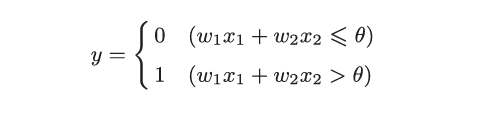输入信号的权重是固有，权重越大，对应的输入信号就越重要。
ps：权重可以为负值。

## 二，感知机算法有什么用

权重和偏置是参数。
给定一个输入，输出一个既定的值。

## 三， 感知机算法如何实现

以逻辑电路的实现为例，下面的0.5，0.7等数字只是无数情况里的一种情况

简单实现：
简单的与门的实现：

def AND(x1, x2):
w1, w2, theta = 0.5, 0.5, 0.7
temp = w1 * x1 + w2 * x2
if temp > theta:
return 1
else:
return 0

print(AND(0, 0))
print(AND(0, 1))
print(AND(1, 0))
print(AND(1, 1))


导入权重和偏置
将θ用-b替换，表达式变为：b被称为偏置，w1，w2是权重。

这里把−θ 命名为偏置b，但是请注意，偏置和权重w1、w2的作用是不
一样的。具体地说，w1和w2是控制输入信号的重要性的参数，而偏置是调整神经元被激活的容易程度（输出信号为1 的程度）的参数。比如，若b 为0.1，则只要输入信号的加权总和超过0.1，神经元就会被激活。但是如果b为−20.0，则输入信号的加权总和必须超过20.0，神经元才会被激活。像这样，
偏置的值决定了神经元被激活的容易程度。另外，这里我们将w1和w2称为权重，将b 称为偏置，但是根据上下文，有时也会将b、w1、w2这些参数统称为权重。

与门的实现：

# coding=utf-8
import numpy as np

def AND(x1, x2):
x = np.array([x1, x2])
w = np.array([0.5, 0.5])
b = -0.7
temp = np.sum(x * w) + b
if temp > 0:
return 1
else:
return 0

print(AND(0, 0))
print(AND(0, 1))
print(AND(1, 0))
print(AND(1, 1))



与非门的实现：

def NAND(x1, x2):  # 与非门
x = np.array([x1, x2])
w = np.array([-0.5, -0.5])
b = 0.7
temp = np.sum(x * w) + b
if temp > 0:
return 1
else:
return 0


或门的实现

def OR(x1, x2):  # 或门
x = np.array([x1, x2])
w = np.array([0.5, 0.5])
b = -0.3
temp = np.sum(x * w) + b
if temp > 0:
return 1
else:
return 0


## 四，感知机算法的局限性

上面实现了与门，与非门和或门，但是无法实现异或门。
先以能实现的或门为例：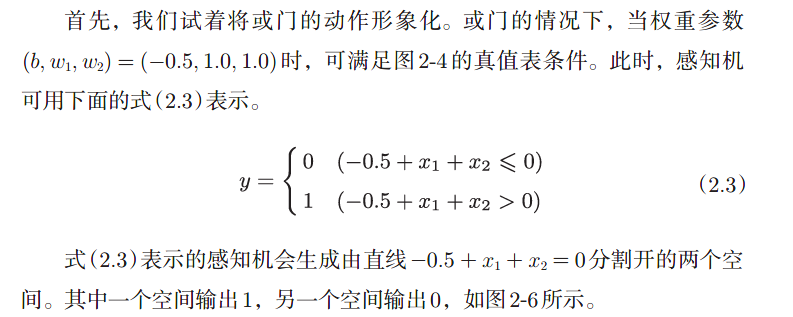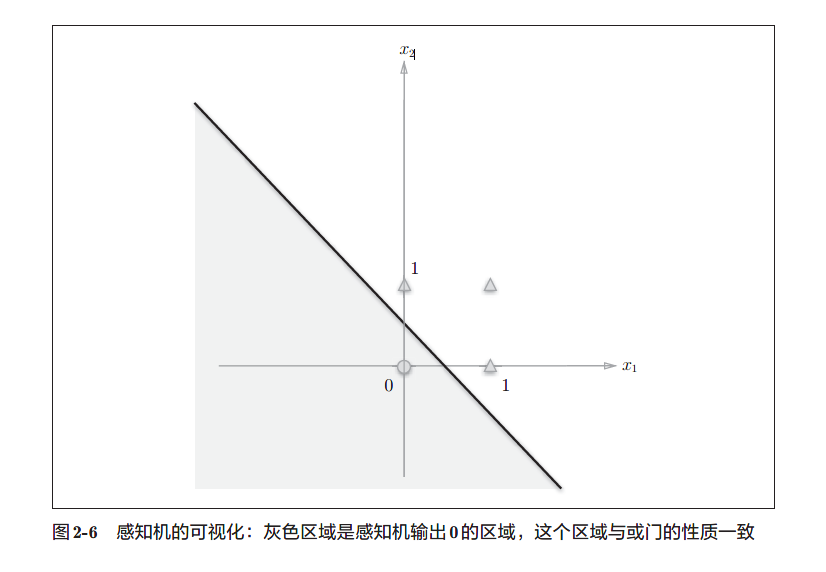或门在(x1,x2) = (0, 0) 时输出0，在(x1,x2) 为(0, 1)、(1, 0)、(1, 1) 时输出1。图2-6 中，○表示0，△表示1。如果想制作或门，需要用直线将图2-6
中的○和△分开。实际上，刚才的那条直线就将这4 个点正确地分开了。

而异或门呢？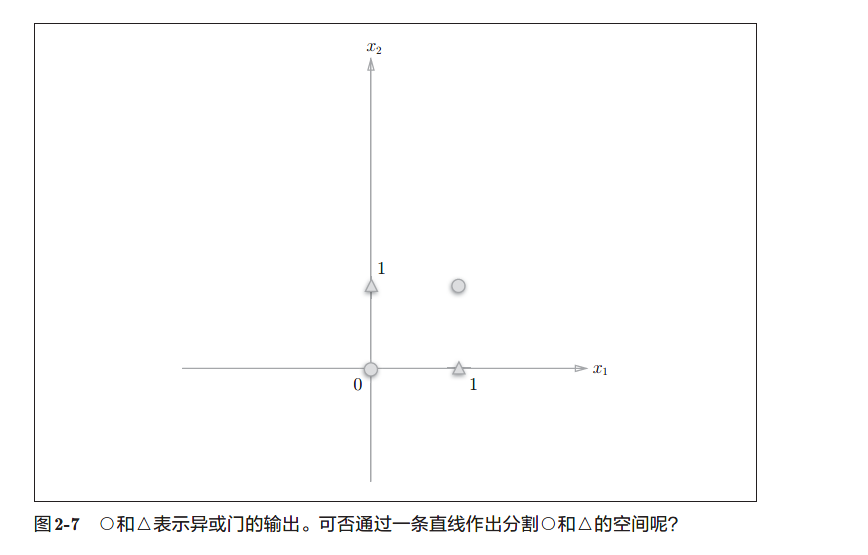显然，用一条直线无法分开4个点。

感知机的局限性在于它只能表示一条直线分开的空间。

## 五，线性和非线性

异或门的情况可以使用一条曲线分开4个点。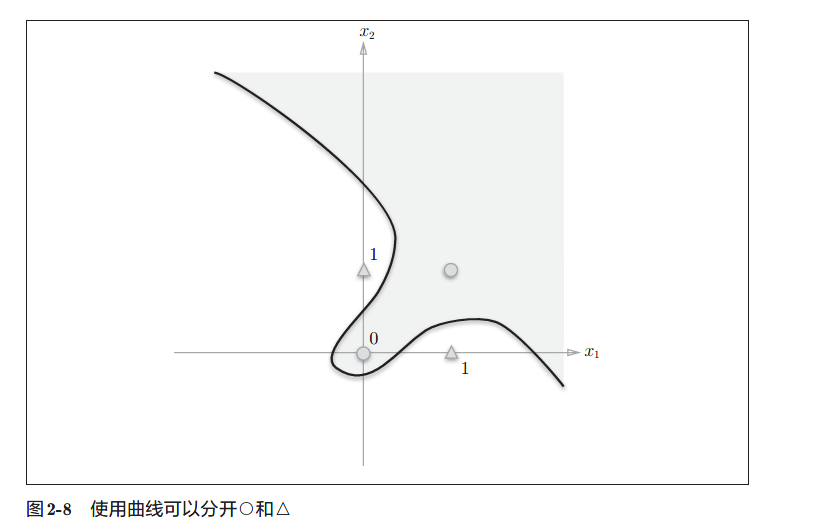由曲线分割开的空间被称为非线性空间
由直线分割开的空间被称为线性空间

## 六，多层感知机

讲到的感知机的局限性，严格地讲，应该是“单层感知机无法
表示异或门”或者“单层感知机无法分离非线性空间”。

通过**组合感知机（叠加层）**就可以实现异或门。

异或门可以使用通过组合与门、与非门、或门来实现。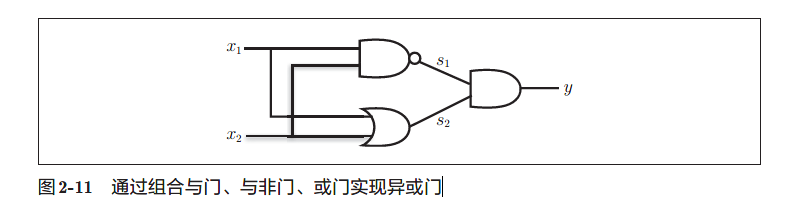def XOR(x1, x2):
s1 = NAND(x1, x2)
s2 = OR(x1, x2)
y = AND(s1, s2)
return y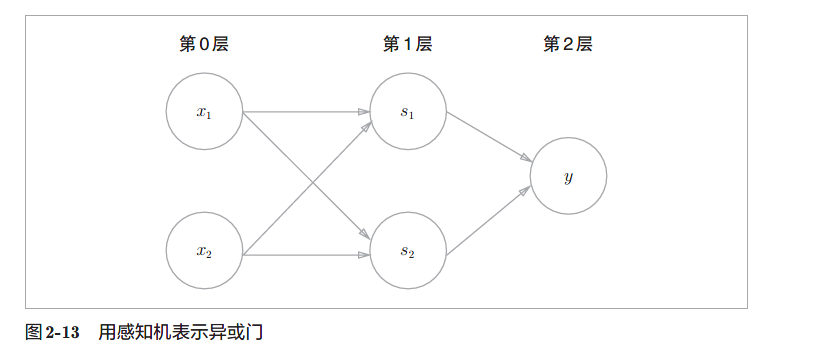如图所示，异或门是一种多层结构的神经网络。这里，将最左边的一列称为第0 层，中间的一列称为第1 层，最右边的一列称为第2 层。
图2-13 所示的感知机与前面介绍的与门、或门的感知机形状不
同。实际上，与门、或门是单层感知机，而异或门是2 层感知机。

叠加了多层的感知机也称为多层感知机（multi-layered perceptron ）。

图2-13 中的感知机总共由3 层构成，但是因为拥有权重的层实质
上只有2层
（第0层和第1层之间，第1层和第2层之间），所以称
为“2层感知机”。不过，有的文献认为图2-13的感知机是由3层
构成的，因而将其称为“3层感知机”。

记住：

感知机通过叠加层能够进行非线性的表示

知识点总结
•感知机是具有输入和输出的算法。给定一个输入后，将输出一个既
定的值。
•感知机将权重和偏置设定为参数。
•使用感知机可以表示与门和或门等逻辑电路。
•异或门无法通过单层感知机来表示。
•使用2层感知机可以表示异或门。
•单层感知机只能表示线性空间，而多层感知机可以表示非线性空间。
•多层感知机（在理论上）可以表示计算机。

展开全文• ## 感知机算法详解

千次阅读 多人点赞 2019-09-03 23:08:27
今天小编给大家带来感知机算法的详解。感知机算法由Rosenblatt在1957年提出，是一类简单的线性判别算法，通过扩展又可以与许多其他算法密切相关。如逻辑回归模型、支持向量机、前馈神经网络（多层感知机）、线性判别...

今天想写一下感知机算法的详解。感知机算法由Rosenblatt在1957年提出，是一类简单的线性判别算法，通过扩展又可以与许多其他算法密切相关。如逻辑回归模型、支持向量机、前馈神经网络（多层感知机）、线性判别分析等。因此感知机算法尽管很少单独使用，但它对于理解其他模型和算法非常有用，很适合作为开始机器学习的一个切入点，同时也是建立知识体系的一个枢纽。

本文首先简要介绍感知机，然后讲解感知机的模型、策略、算法，最后分析感知机算法与各个算法之间的联系并做出总结。

## 感知机能做什么

感知机是一种二分类模型，其输入为样本的特征向量，输出为样本的类别，取+1和-1二值。要得到正确的模型，感知机要求数据集本身线性可分：
在二维平面上，线性可分意味着能用一条直线将正、负样本分开；
在三维空间中，线性可分意味着能用一个平面将正、负样本分开；
在n维空间中，线性可分意味着能用n-1维超平面将正、负样本分开。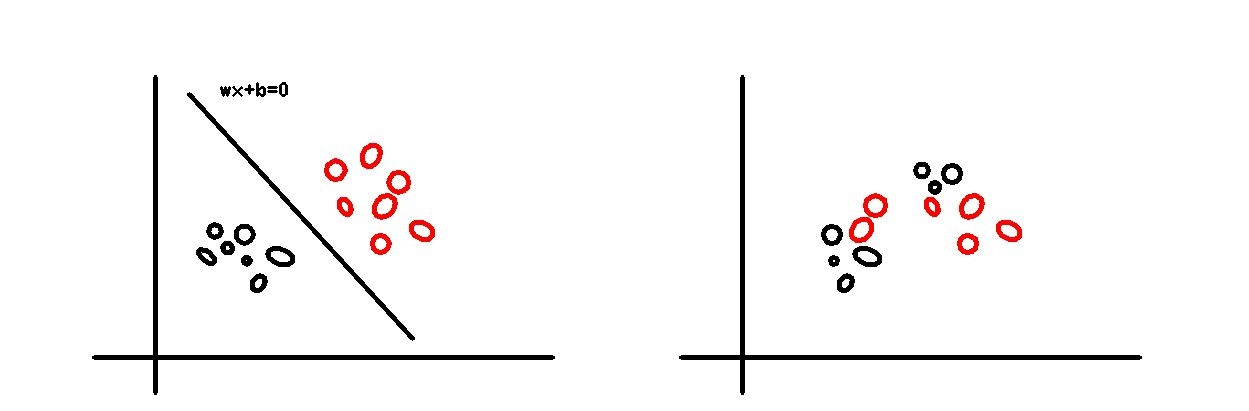图1 二维平面上的线性可分与线性不可分

为了便于应用感知机算法，我们有时会使用一些技巧，使得线性不可分的样本在某些变换下成为线性可分。这些技巧包括将样本分离到更高维空间（图2）或投影到特定的方向（图3）等，这是另一大类方法，本文主要讲解一般的感知机，此处不详细展开讨论。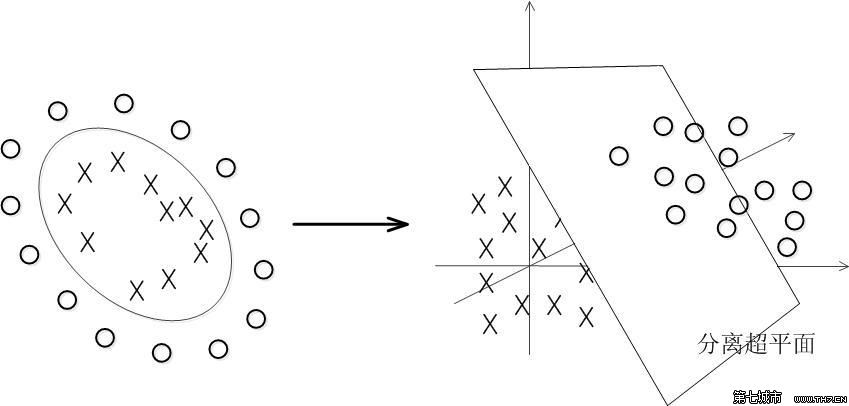图2 分离到高维空间实现线性可分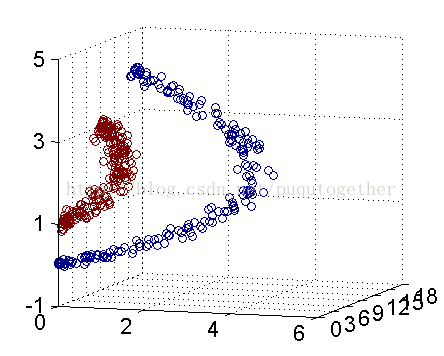图3 投影特定方向实现线性可分

## 感知机模型

• 定义
设输入空间（特征空间）为 X ⊆ R n X\subseteq\R^n ,输出空间为 Y = { − 1 , + 1 } Y=\{-1,+1\}
输入 x ∈ X x\in X 为实例的特征向量 输出 y ∈ Y y\in Y 为实例的类别
由输入空间到输出空间的如下函数称为感知机
f ( x ) = s i g n ( w x + b ) f(x) = sign(wx+b) 其中 w w b b 为模型参数， w ∈ R n w\in\R^n 称为权值， b ∈ R b\in\R 称为偏置。 s i g n sign 是符号函数。

感知机模型有直观的几何解释：线性方程 w x + b = 0 wx+b=0 对应于分离超平面 S S ,其中 w w S S 的法向量， b b S S 的截距。求解感知机，就是要解出 w w b b ,得到能正确分离所有正负样本的超平面 S S (见图1).

## 感知机策略

为找出正确的分离超平面、确定感知机模型参数，需要确定一个学习策略。在监督学习中，使用某种策略即是选用相应的损失函数。
考虑以在S划分下误分类点的总数作为损失函数，该函数可以自然地监督感知机的分类性能，但它不是参数 w w b b 的连续可导函数，不易优化。如图4，当超平面在空间中由 S 1 S1 连续变化至 S 3 S3 时，相应的法向量 w w 也连续变化，而误分类点数量则是不连续的。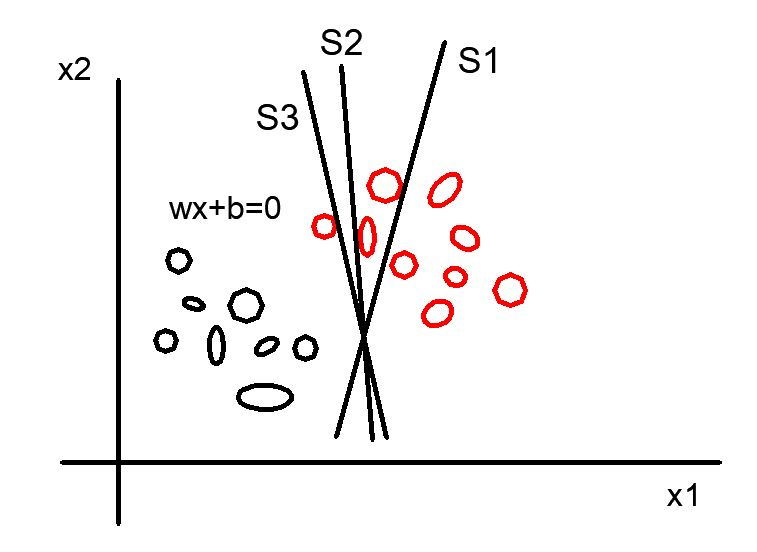图4 误分类点个数不连续变化

为此感知机采用的损失函数为:误分类点到超平面 S S 的总距离，该函数对 w w b b 连续可导。
单个点 x i x_i 到超平面 S S 的距离为 − 1 ∥ w ∥ ∣ w x i + b ∣ -\frac{1}{\Vert w \Vert}\vert wx_i+b \vert ,

对于误分类点数据 ( x i , y i ) (x_i,y_i) ,有 − y i ( w x i + b ) > 0 -y_i(wx_i+b)>0 ,因为 y i y_i w x i + b wx_i+b 异号

设超平面 S S 的误分类集合为 M M ,则所有误分类点到超平面 S S 的总距离为 − 1 ∥ w ∥ ∑ x i ∈ M y i ( w x i + b ) -\frac{1}{\Vert w \Vert}\sum_{x_i\in M}y_i(wx_i+b) 不考虑式中的 − 1 ∥ w ∥ -\frac{1}{\Vert w \Vert} ,即得到感知机学习的损失函数。

• 损失函数
给定训练集 T = { ( x 1 , y 1 ) , ( x 2 , y 2 ) , . . . , ( x N , y N ) } T=\{(x_1,y_1),(x_2,y_2),...,(x_N,y_N)\} ,其中 x i ∈ X = R n x_i\in X=\R^n ,
y i ∈ Y = { − 1 , + 1 } , i = 1 , 2 , . . . , N y_i\in Y=\{-1,+1\},i=1,2,...,N .感知机学习的损失函数为
L ( w , b ) = − ∑ x i ∈ M y i ( w x i + b ) L(w,b)=-\sum_{x_i\in M}y_i(wx_i+b)

易知损失函数是非负的，误分类点越少，误分类点离超平面越近，损失函数值就越小。如果没有误分类点，则损失函数值时0.感知机学习的策略是在假设空间中选取使损失函数取值最小的模型参数。

## 感知机学习算法

### 原始形式

目标：感知机学习算法的原始形式是求解最优化问题 m i n w , b L ( w , b ) = − ∑ x i ∈ M y i ( w x i + b ) {min}_{w,b}L(w,b)=-\sum_{x_i\in M}y_i(wx_i+b) 其中 M M 为误分类点的集合。
算法：误分类驱动的随机梯度下降法首先选取初始超平面 w 0 , b 0 w_0,b_0 ,然后计算在该参数取值处的梯度，用梯度下降法不断的极小化目标函数。极小化过程中，不是一次使用 M M 中所有误分类点计算梯度，而是一次随机选取一个误分类点使其梯度下降。设误分类点集合 M M 是固定的，则损失函数的梯度为： ∇ w L ( w , b ) = − ∑ x i ∈ M y i x i \nabla_w L(w,b)=-\sum_{x_i\in M}y_ix_i ∇ b L ( w , b ) = − ∑ x i ∈ M y i \nabla_bL(w,b)=-\sum_{x_i\in M}y_i 由于采用随机梯度下降，一次随机选取一个误分类点 ( x i , y i ) (x_i,y_i) , w , b w,b 的更新公式为： w ← w + η y i x i , b ← b + η y i w\leftarrow w+\eta y_ix_i, b\leftarrow b+\eta y_i 式中 η （ 0 ≤ η ≤ 1 ） \eta（0\leq\eta\leq1） 称为学习率。整理称为算法形式如下：

• 算法1
输入：线性可分的训练数据集 T = { ( x 1 , y 1 ) , ( x 2 , y 2 ) , . . . , ( x N , y N ) } T=\{(x_1,y_1),(x_2,y_2),...,(x_N,y_N)\} ,
其中 x i ∈ X = R n x_i\in X=\R^n , y i ∈ Y = { − 1 , + 1 } , i = 1 , 2 , . . . , N y_i\in Y=\{-1,+1\},i=1,2,...,N . 学习率 η （ 0 ≤ η ≤ 1 ） \eta（0\leq\eta\leq1）
输出：参数 w , b w,b ，感知机模型 f ( x ) = s i g n ( w x + b ) f(x)=sign(wx+b)
(1)选取初值 w 0 ， b 0 w_0，b_0
(2)在训练集中选取数据 ( x i , y i ) (x_i,y_i)
(3)如果 y i ( w x i + b ) ≤ 0 y_i(wx_i+b)\leq0 w ← w + η y i x i , b ← b + η y i w\leftarrow w+\eta y_ix_i, b\leftarrow b+\eta y_i
(4)转至（2），直至训练集中没有误分类点。
每次根据 ( x i , y i ) (x_i,y_i) 调整 w , b w,b 时，分离超平面将向该误分类点移动，以减少该误分类点与超平面的距离，越过该误分类点使其正确分类。

感知机学习算法的收敛性有定理保证，这里不再详细阐述，有兴趣的读者可以查阅相关资料。

### 对偶形式

感知机学习算法的原始形式是每一步直接更新参数 w , b w,b ,经过n步达到收敛。对偶形式的基本想法是，将 w w b b 表示为实例 x i x_i 和标记 y i y_i 的线性组合的形式，通过求解其系数而求得 w w b b .假设初值 w 0 , b 0 w_0,b_0 均为0，对误分类点 ( x i , y i ) (x_i,y_i) 通过 w ← w + η y i x i w\leftarrow w+\eta y_ix_i b ← b + η y i b\leftarrow b+\eta y_i 逐步修改 w , b w,b ,设收敛过程中，第 i i 个点共修改了 n i n_i 次，则 w , b w,b 关于 ( x i , y i ) (x_i,y_i) 的增量分别是 α i y i x i \alpha_i y_ix_i α i y i \alpha_iy_i ,其中 α i = n i η \alpha_i=n_i\eta .对N个样本点上 w , b w,b 的增量求和，有 w = ∑ i = 1 N α i y i x i w=\sum_{i=1}^N\alpha_iy_ix_i b = ∑ i = 1 N α i y i b=\sum_{i=1}^N\alpha_iy_i 从而感知机可以写成由系数 α i , b \alpha_i,b 表示的形式 f ( x ) = s i g n ( ∑ j = 1 N α j y j x j ⋅ x + b ) f(x)=sign(\sum_{j=1}^N\alpha_jy_jx_j\cdot x+b) ，上述过程整理为算法2.

• 算法2
输入：线性可分的训练数据集 T = { ( x 1 , y 1 ) , ( x 2 , y 2 ) , . . . , ( x N , y N ) } T=\{(x_1,y_1),(x_2,y_2),...,(x_N,y_N)\} ,其中 x i ∈ X = R n x_i\in X=\R^n , y i ∈ Y = { − 1 , + 1 } , i = 1 , 2 , . . . , N y_i\in Y=\{-1,+1\},i=1,2,...,N . 学习率 η （ 0 ≤ η ≤ 1 ） \eta（0\leq\eta\leq1）
输出：参数 α , b \alpha,b ，感知机模型 f ( x ) = s i g n ( ∑ j = 1 N α j y j x j ⋅ x + b ) f(x)=sign(\sum_{j=1}^N\alpha_jy_jx_j\cdot x+b) ，其中 α = ( α 1 , α 2 , . . . , α N ) T \alpha=(\alpha_1,\alpha_2,...,\alpha_N)^T
(1)取初值 α ← 0 , b ← 0 \alpha \leftarrow0,b\leftarrow0
(2)在训练集中选取数据 ( x i , y i ) (x_i,y_i)
(3)如果 y i ( ∑ j = 1 N α j y j x j ⋅ x i + b ) ≤ 0 y_i(\sum_{j=1}^N\alpha_jy_jx_j\cdot x_i+b)\leq0 α i ← α i + η \alpha_i\leftarrow\alpha_i+\eta b ← b + η y i b\leftarrow b +\eta y_i
(4)转至（2）中直到没有误分类数据。

对偶形式算法的特点是， α i \alpha_i 每步更新差值固定， α i \alpha_i 越大更新次数越多，意味着 ( x i , y i ) (x_i,y_i) 离分离超平面越近，对学习结果的影响也最大。
对偶形式中训练实例仅以内积形式出现，可以预先将实例间的内积计算出来并存储为矩阵，即Gram矩阵。 G = [ x i ⋅ x j ] N × N G=[x_i\cdot x_j]_{N\times N} Gram矩阵是 N × N N\times N 的对称矩阵

## 感知机与其他算法

感知机模型简单、易学习，是基本的机器学习模型，很多机器学习模型是在感知机模型的基础上变化扩展而得到的。
感知机模型由于其简单，在应用时也有一定的局限，主要体现在以下几点。

• 感知机只能处理线性可分的数据集，线性不可分的数据集会引起分离超平面的震荡而无法收敛。
• 感知机是误分类驱动的模型，模型的解不唯一。离群点对结果影响很大。
• 感知机的判别模型由符号函数给出，无法表示更复杂的非线性映射。

为解决以上的局限性，其他算法从不同方向对感知机模型进行了扩展。

### 感知机与支持向量机

• 相同点
感知机与支持向量机都是求解能正确划分样例的超平面

• 不同点
感知机是误分类驱动的，其损失函数来源于误分类点到分离超平面的距离之和。数据集线性可分时，能正确划分样例的超平面有无穷多个。
支持向量机不仅要求正确划分正负样例，还要求正负样例间隔最大，在最大间隔约束下，划分超平面（的法向量）有唯一解。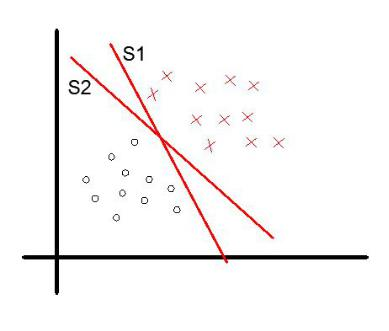图5 感知机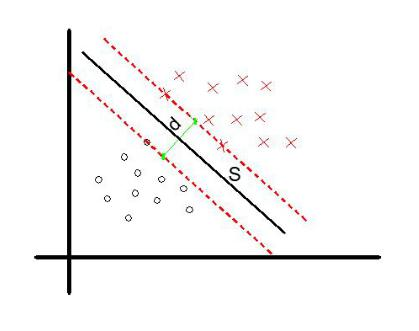图6 支持向量机

感知机优化目标： m i n w , b L ( w , b ) = − ∑ x i ∈ M y i ( w x i + b ) {min}_{w,b}L(w,b)=-\sum_{x_i\in M}y_i(wx_i+b) 支持向量机优化目标： m a x w , b d {max}_{w,b} d

### 感知机与逻辑回归

感知机与逻辑回归都是判别模型，都是对线性运算的结果进行判别，区别是采用了不同判别方式。

• 感知机用符号函数进行判别： w ⋅ x + b → f ( x ) = s i g n ( w ⋅ x + b ) w\cdot x+b \rightarrow f(x)=sign(w\cdot x+b)
• 逻辑回归用对数几率进行判别： w ⋅ x + b = l o g p 1 − p → p = 1 1 + e − ( w ⋅ x + b ) w\cdot x+b=log \frac{p}{1-p}\rightarrow p=\frac{1}{1+e^{-(w\cdot x+b)}}
如果把神经元看成是对线性输入做非线性变换并输出的算法部件，则感知机和逻辑回归可以看成是两种不同的神经元，即采用了不同的非线性变换。

### 感知机与神经网络

当把感知机看成单个神经元时，该神经元的堆叠就形成了神经网络。最常用的前馈神经网络就是感知机分层堆叠而形成的，也叫多层感知机MLP.感知机的激活函数是阶跃函数（符号函数）： f ( x ) = s i g n ( x ) = { 1 , x > 0 − 1 , x ≤ 0 f(x)=sign(x)=\begin{cases}1, x>0\\-1,x\leq0\end{cases}
目前常用神经网络的激活函数是线性整流函数(ReLU): f ( x ) = R e L U ( x ) = { x , x > 0 − 1 , x ≤ 0 f(x)=ReLU(x)=\begin{cases}x, x>0\\-1,x\leq0\end{cases}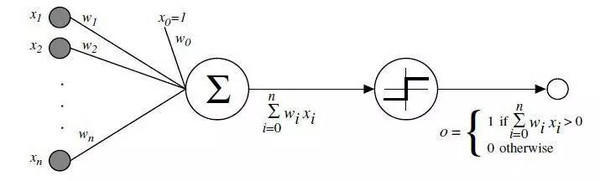图7 感知机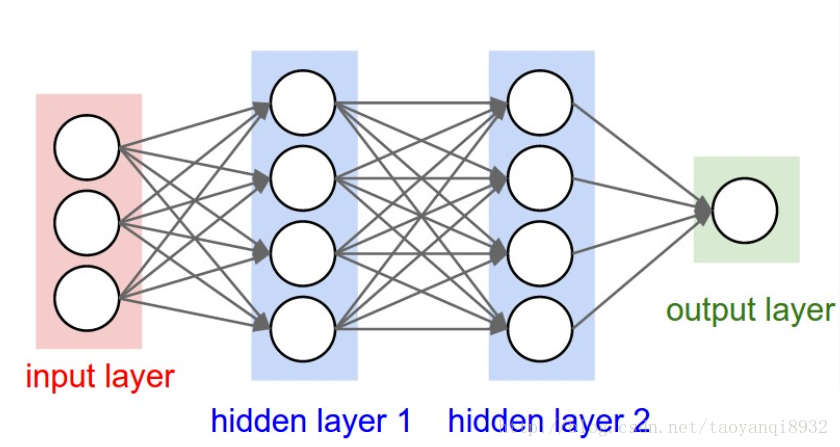图8 MLP(图中每个神经元相当于一个感知机)

关于感知机算法的理论部分小编就介绍这么多，它的实现可以使用常用机器学习开源库sklearn中的模块MLPClassifier来完成。感兴趣的读者不妨去试试哦。

展开全文机器学习
• 词性标注的python实现-基于平均感知机算法-附件资源
• 文章目录经典分类算法——感知机算法1 感知机算法思想：错误修正2 感知机算法（原始形式）：形式化表示3 感知机算法（对偶形式）：形式化表示4 感知机算法：随机梯度下降（SGD）5 感知机算法：一种变形6 感知器算法...

# 经典分类算法——感知机算法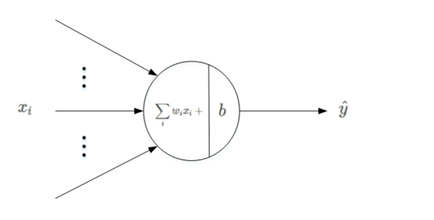​ 二维分类问题是一个经典的机器学习问题，感知机算法(Perception Approach)是解决该问题的经典算法之一。虽然其本身是一类简单的线性判别算法，但是通过扩展又可以与许多其他算法密切相关。因此感知机算法尽管很少单独使用，但它对于理解其他模型和算法非常有用，是建立知识体系的一个枢纽。

## 1 感知机算法思想：错误修正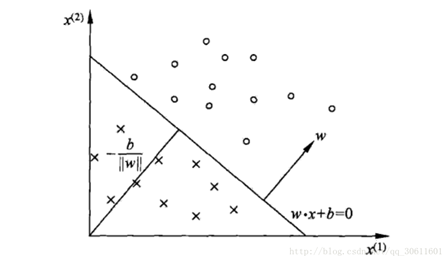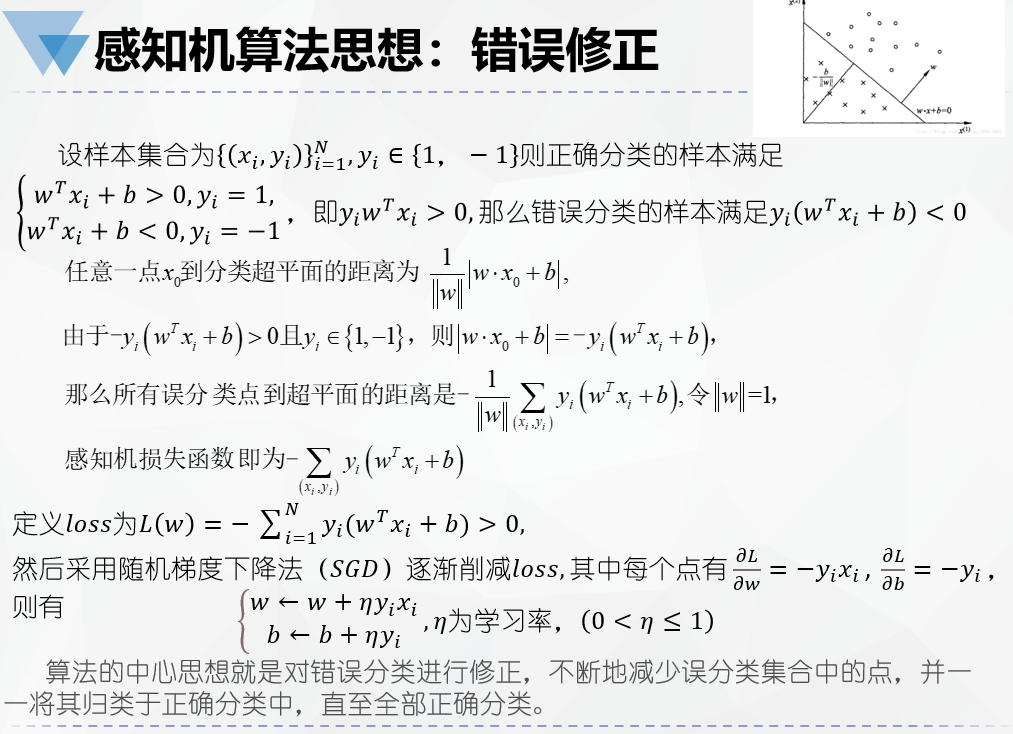## 2 感知机算法（原始形式）：形式化表示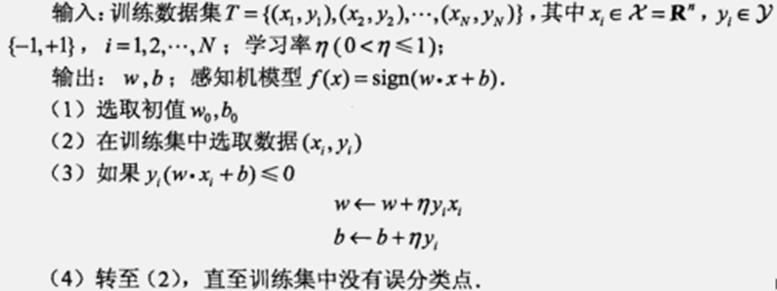​ 当一个点被误分类位于分类超平面错误一侧时，则调整w，b的值，使分类超平面向该误分类点的一侧移动，以减少该误分类点与超平面之间的距离，直至分类超平面越过该误分类点使其被正确分类。

## 3 感知机算法（对偶形式）：形式化表示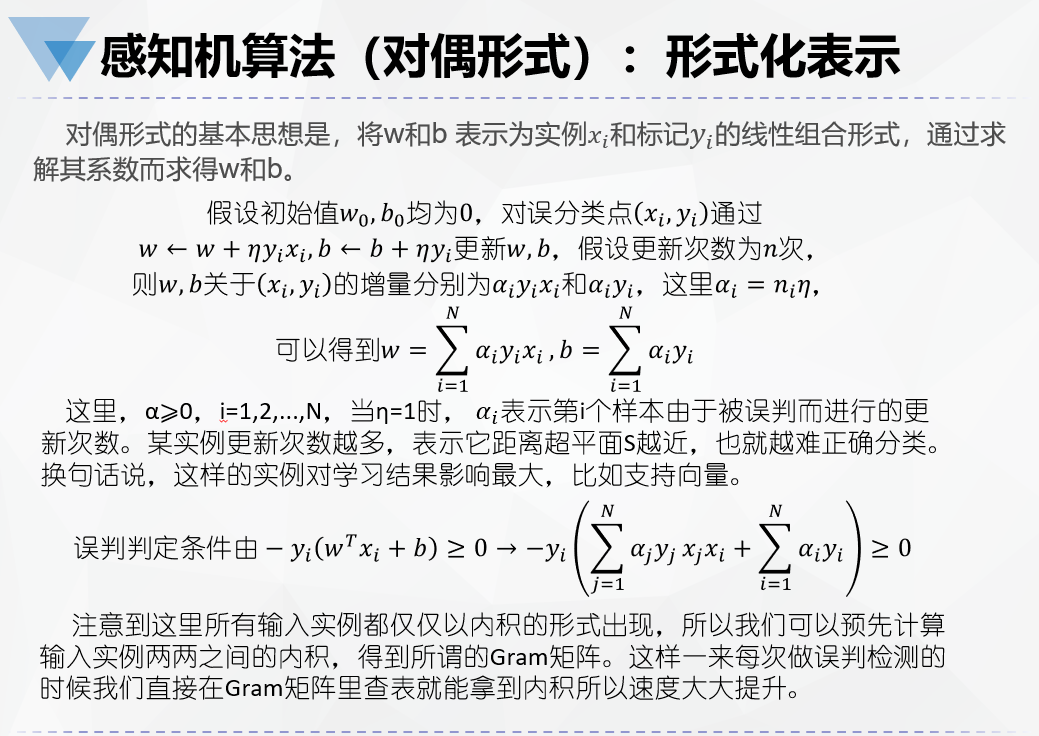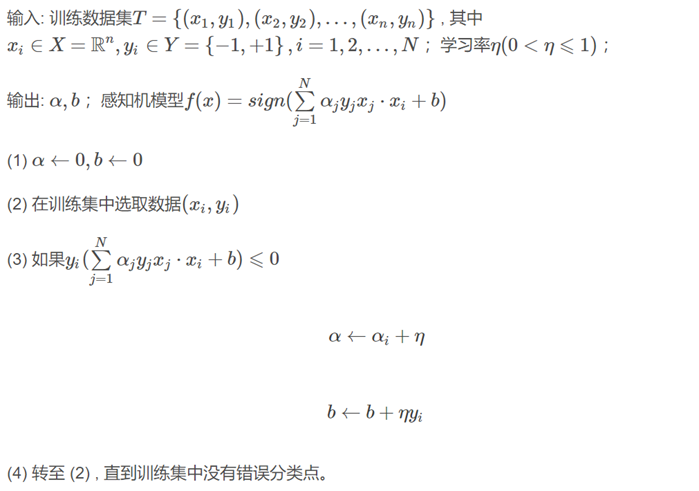## 4 感知机算法：随机梯度下降（SGD）

​ 梯度的本意是一个向量，表示某一函数在该点处的方向导数沿着该方向取得最大值，即函数在该点处沿着该方向（梯度的方向）变化最快，变化率最大（为该梯度的模）。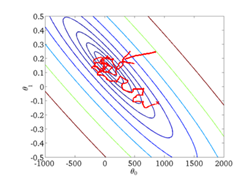随机梯度下降法的求解过程可以概括如下：

1-随机一个初始值,在多元线性回归中，我们随机一组 ,带入到损失函数中,得到一个初始点。

2-让这个点按照负梯度的方向运动，更新参数𝜃

3-迭代第二步,当迭代此处达到某一个数，或者上一步和这一步的结果误差小于某个数,就认为是最优解了，停止迭代。迭代次数和最小误差值都是可以设置的.## 5 感知机算法：一种变形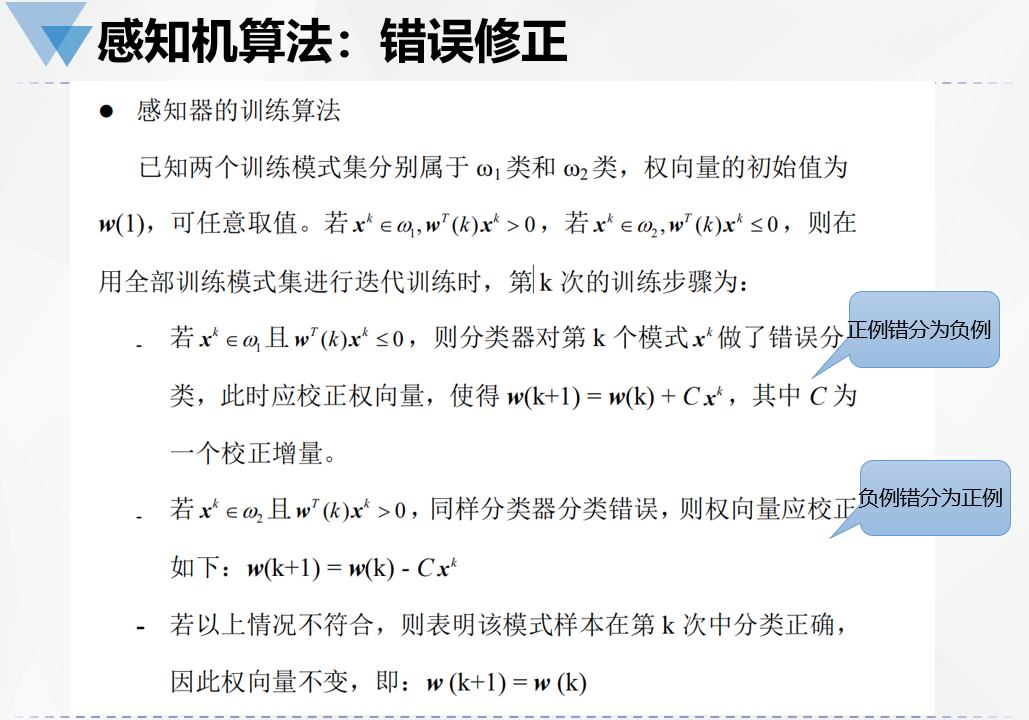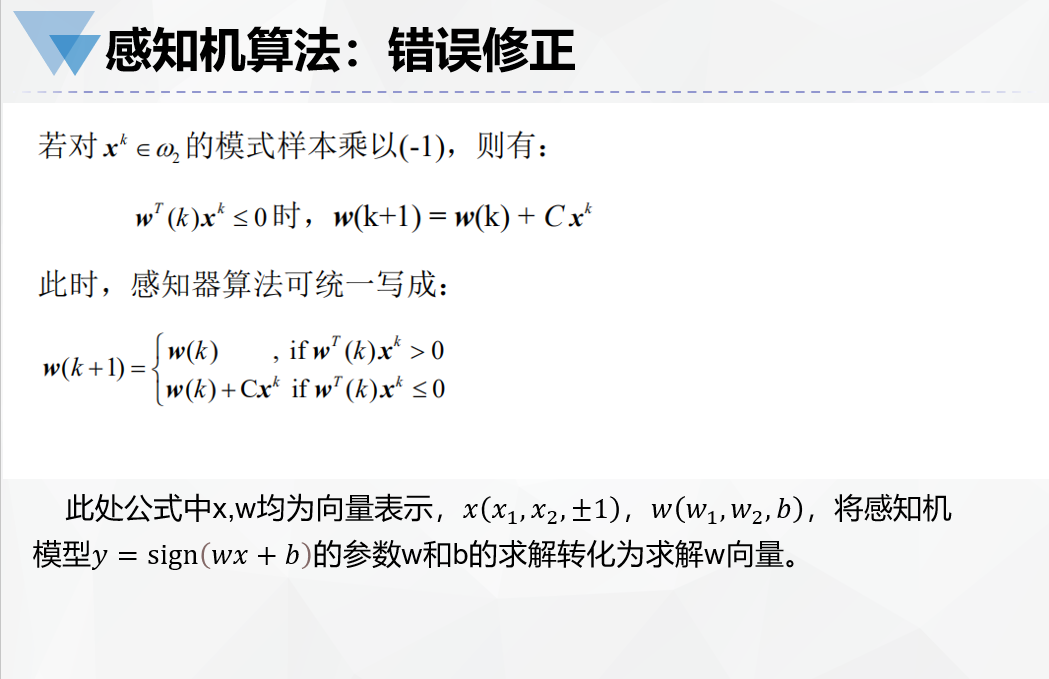## 6 感知器算法：示例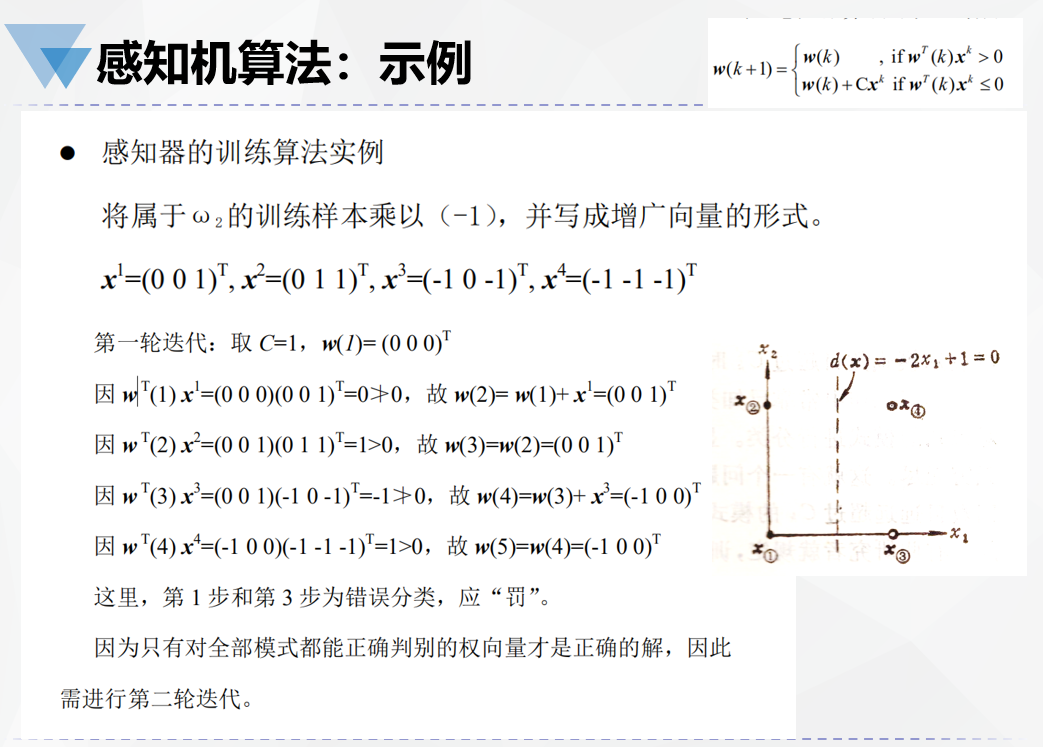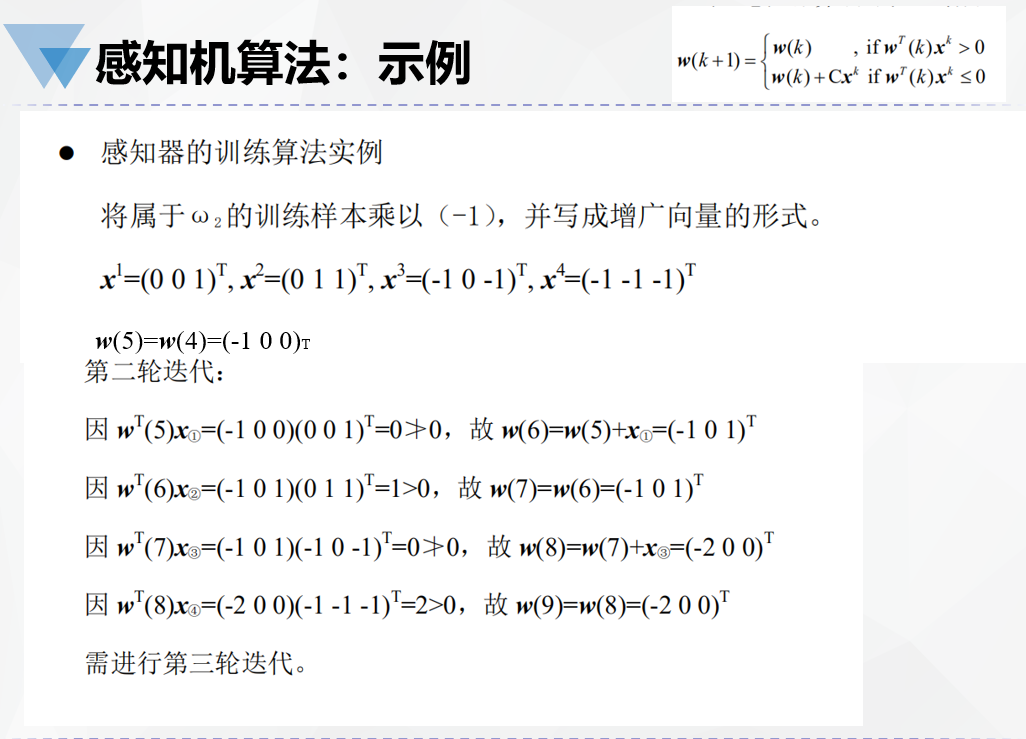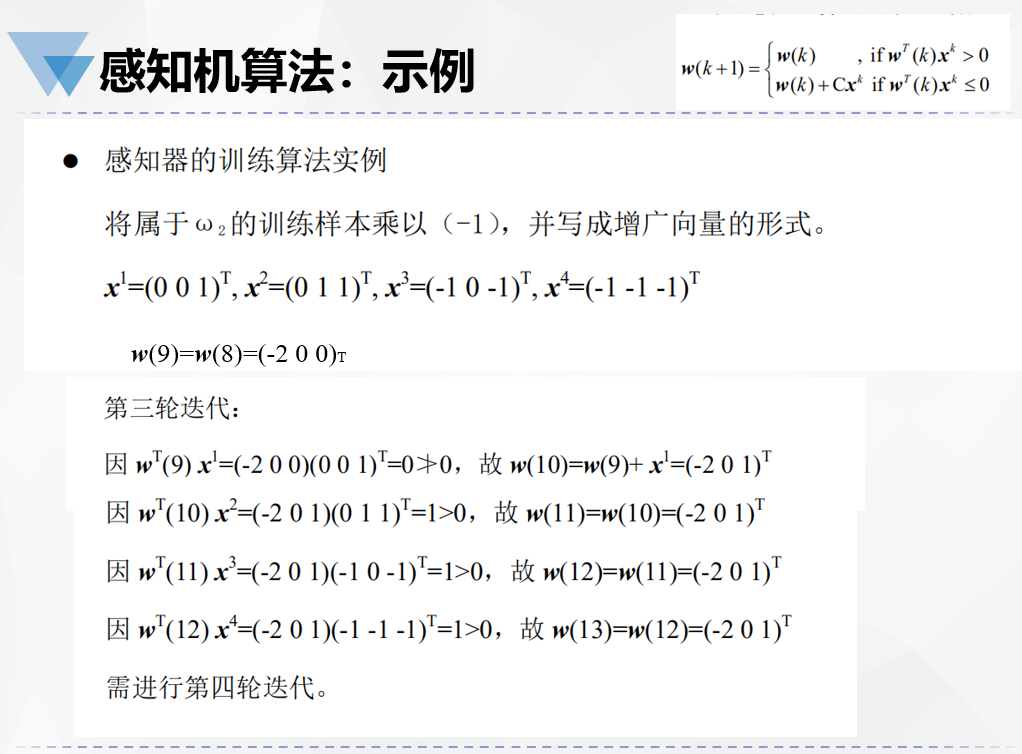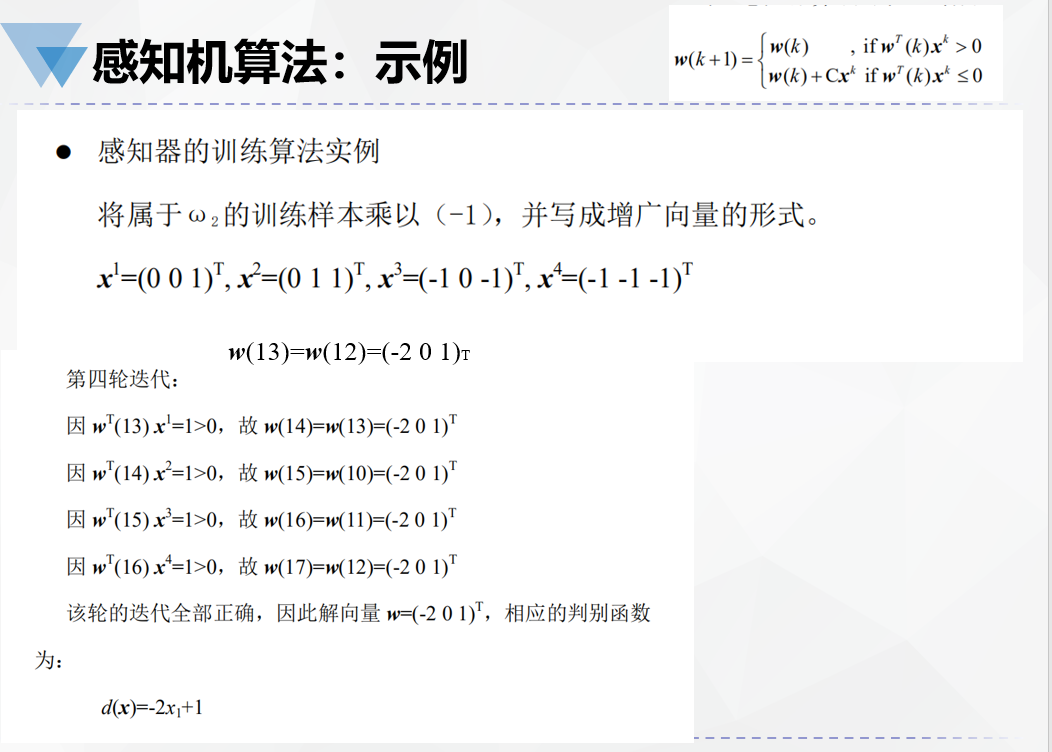## 7 感知器算法：拓展——多分类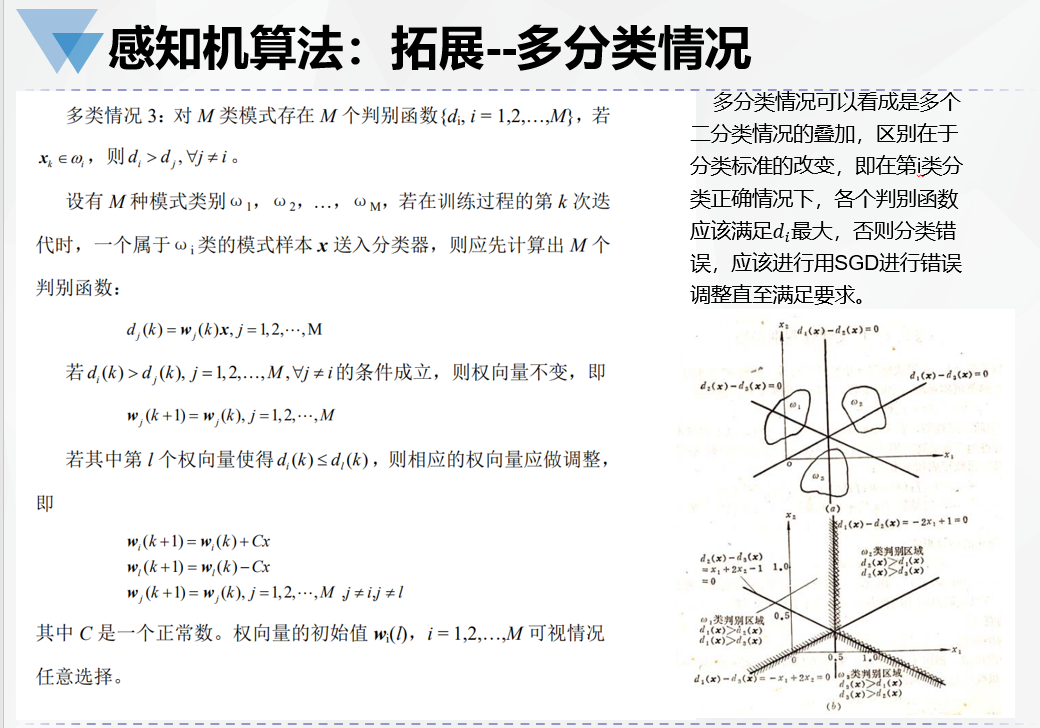## 8 感知机算法：小结

• 感知机算法是一种朴素简单的算法，通过随机梯度下降不断修正错误直至满足分类正确或达到要求。
• 感知机算法是后面许多分类算法的鼻祖，比如SVM、深度神经网络。
• 单层感知机只能对线性可分的向量进行分类，两层及以上的感知机可以模拟任意的函数，后来逐渐演变发展为深度学习中的神经网络。
展开全文分类
• 该代码包括感知机学习算法和对偶形式的感知机学习算法，应用在二分类的问题上，整体上表现出不错的效果
•matlab 机器学习
• 1，概述 1.1，定义 感知机（Perceptron）： ... 感知机学习算法具有简单而易于实现的优点，分为原始形式和对偶形式； 感知机的缺点：我们在不知道数据的情况下（是否线性可分）PLA就不会停下来，这个时候机器学习
• 一、感知机的定义 感知机是二分类的线性分类模型，输入多个信号，输出一个信号（类别），属于判别模型。 二、感知机模型 感知机模型的公式： 其中w表示输入信号在模型公式中的权重，b输入信号在...机器学习 python
• 感知机算法是一个比较古老的机器学习算法了，是Rosenblatt在1957年提出的，是神经网络和支持向量机的基础。感知机算法只能解决线性分类模型。 算法原理 1. 感知机算法的原始形式 感知机模型可以表示为：f(x)=sign...
• 资源名：感知机算法_最小平方误差算法_线性SVM算法设计分类器_画出决策面_比较性能_matlab 资源类型：matlab项目全套源码 源码说明： 全部项目源码都是经过测试校正后百分百成功运行的，如果您下载后不能运行可联系...